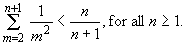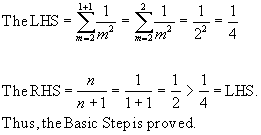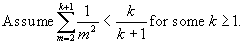Part I. Choose exactly one question (Question 1 or Question 2) in this part to answer.

1. Define a function g: N ® N by the following recurrence, where N denotes the set of natural numbers:
2. g(0) = 1; g(1) = 3; and

g(n) = g(n - 1) + 2 g(n - 2) - 2n + 5, for all n ³ 2.

Prove by induction that g(n) = 2n + n for all n ³ 0.

Solution:

Prove by induction on n ³ 0 that

g(n) = 2n + n.

(Basic Step) Consider the cases of n = 0 and n = 1:

In the case that n = 0: Using the formula, g(0) = 20 + 0 = 1 + 0 = 1, which is equal to the

initial value defined for g(0).

In the case that n = 1: Using the formula, g(1) = 21 + 1 = 2 + 1 = 3, which is equal to the

initial value defined for g(1).

Thus, the formula for g(n) is correct for n = 0 and n = 1.

(Induction Hypothesis) Suppose the formula g(n) = 2n + n is correct for all n in the range

0 £ n £ k, for some k ³ 1.

(Induction Step) Consider n = k + 1. We need to prove g(k+1) = 2k+1 + (k+1) -------(1)

Note that g(k+1) = g(k+1- 1) + 2g(k+1- 2) – 2(k+1) + 5, by applying the recurrence to

g(k+1), since k + 1 ³ 1 + 1 = 2.

= g(k) + 2g(k- 1) – 2k – 2 + 5

= (2k + k) + 2(2k- 1+ k- 1) – 2k + 3, by applying the induction hypothesis to

g(k) and g(k- 1)

= 2k + k + 2k + 2k – 2 – 2k + 3

= 2(2k) + k + 1

= 2k+1 + k + 1

= RHS of (1)

Thus, the induction step is proved. By induction, we proved g(n) = 2n + n for all n ³ 0.

3. Prove by induction that
4.Solution: We use induction on n ³ 1.

(Basic Step) Consider n = 1.(Induction Hypothesis) Consider n = k.

(Induction Step) Consider n = k+1. We need to proveNote that (3) is true because (k + 2)2 > (k + 2)(k +1).

Thus, the induction step is proved. By induction, we proved the problem.

Part II. Choose exactly one question (Question 3 or Question 4) in this part to answer.

5. Let A and B denote two non-empty sets, and let R and S denote two binary relations where R Ì A ´ B and S Ì A ´ B. Answer the following two parts for this question:
1. Suppose both R and R Ç S define a function from A to B. Prove that R Ì S.
2. (This part is independent of Part (a).) Suppose both R and R È S define a function from A to B. Prove that S Ì R.

Solution:

(a) In order to prove R Ì S, let (x, y) Î R ----(1), we need to prove (x, y) Î S ----(2).

Since R Ç S defines a function from A to B, there exists a pair (x, z) Î R Ç S ----(3) for some z Î B; that is, for xÎ A, the function R Ç S maps x to z Î B. From (3), (x, z) Î R Ç S Ì R.

Thus, both (x, y) Î R, from (1), and (x, z) Î R. However, R defines a function from A to B by assumption; thus, x must be mapped under R to a unique element in B, i.e. y = z. Therefore,

(x, y) = (x, z) Î R Ç S, by (3)

Ì S, by the definition of Ç .

Thus, (2) is proved.

1. In order to prove S Ì R, let (x, y) Î S ---(4), we need to prove (x, y) Î R ----(5).

Since R defines a function from A to B, R maps x to some element z Î B, i.e., (x, z) Î R --(6)

Therefore, (x, y) Î S Ì R È S and (x, z) Î R Ì R È S. Since R È S defines a function from A to B, it maps x to a unique element in B, i.e., y = z. Therefore, (x, y) = (x, z) Î R, by (6).

Thus, (5) is proved.

1. Let A = {a, b} denote an alphabet of two distinct symbols. Define a function g: A* ® A* by the following recursive rules:
1. g(l ) = l , where l denotes the empty string.
2. g(a) = b.
3. g(bu) = bg(u) for any u Î A*.
4. g(aau) = g(au) for any u Î A*.
5. g(abu) = bg(bu) for any u Î A*.

Prove by induction that g(anb) = b2, for all n ³ 1.

Solution:

We use induction on n ³ 1 to prove g(anb) = b2.

(Basic Step) When n = 1. In this case,

g(anb) = g(ab)

= g(abl ), because ab = abl

= bg(bl ), Rule (5)

= bbg(l ), Rule (3)

= b2l , Rule (1)

= b2, because b2l = b2.

Thus, the Basis step is proved.

(Induction Hypothesis) Consider n = k.

Assume g(akb) = b2 for some k ³ 1.

(Induction Step) Consider n = k + 1. We need to prove g(ak+1b) = b2 ----(1)

Note that g(ak+1b) = g(aaak- 1b), since k ³ 1 so k + 1 ³ 2

= g(aak- 1b), Rule (4) with u= ak- 1b

= g(akb)

= b2, by the I.H.

Thus, the induction step is proved. By induction, we proved g(anb) = b2 for all n ³ 1.